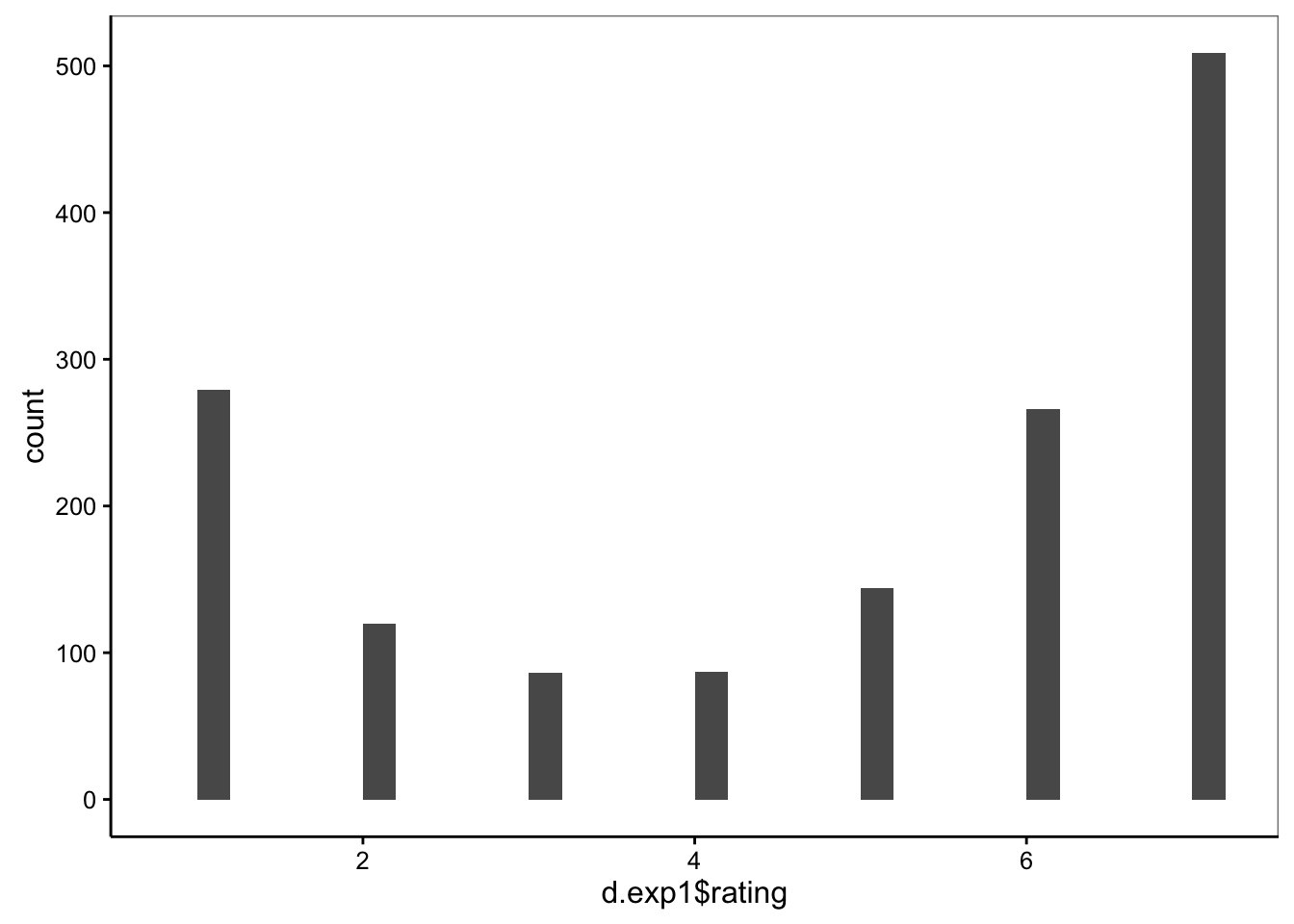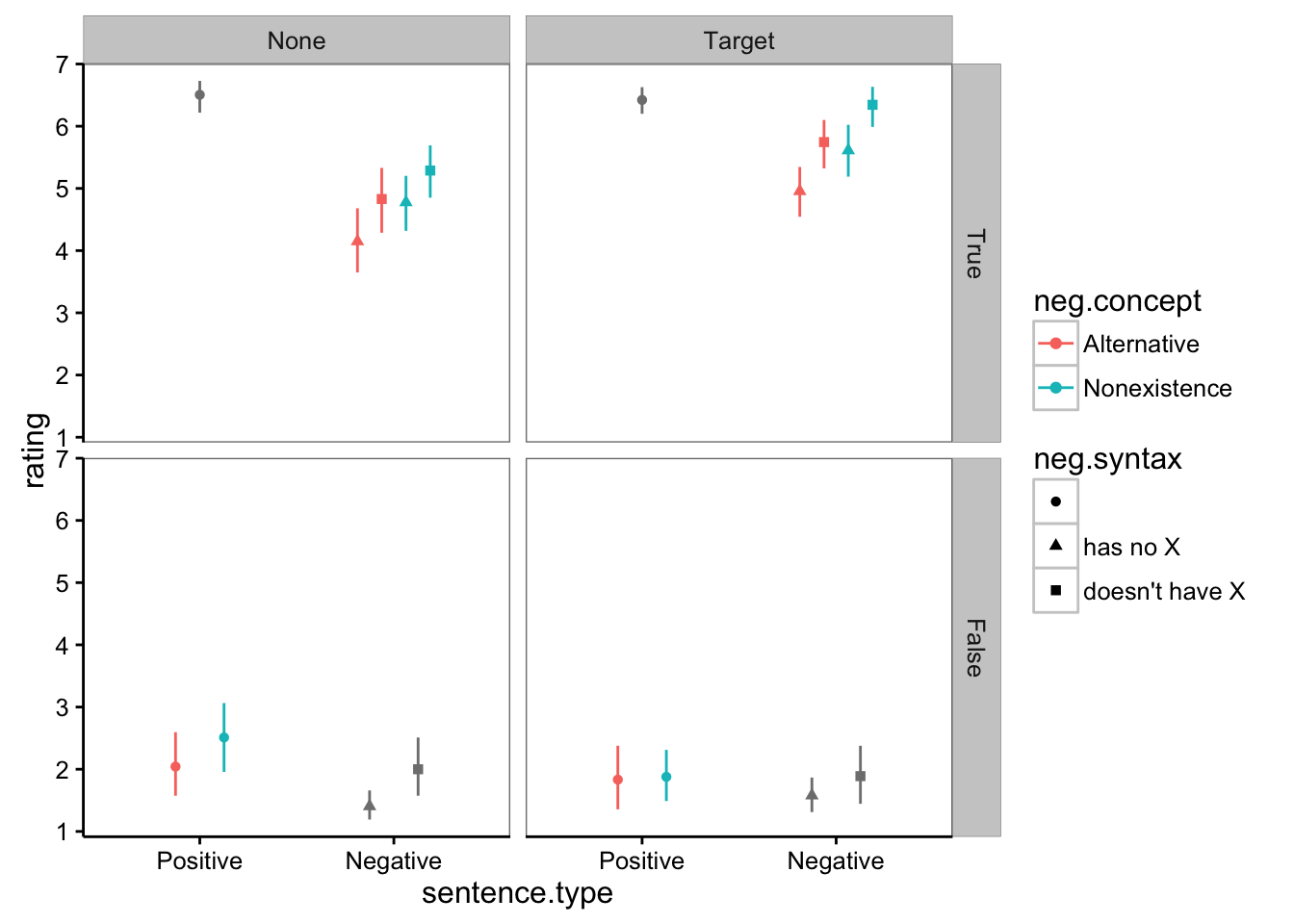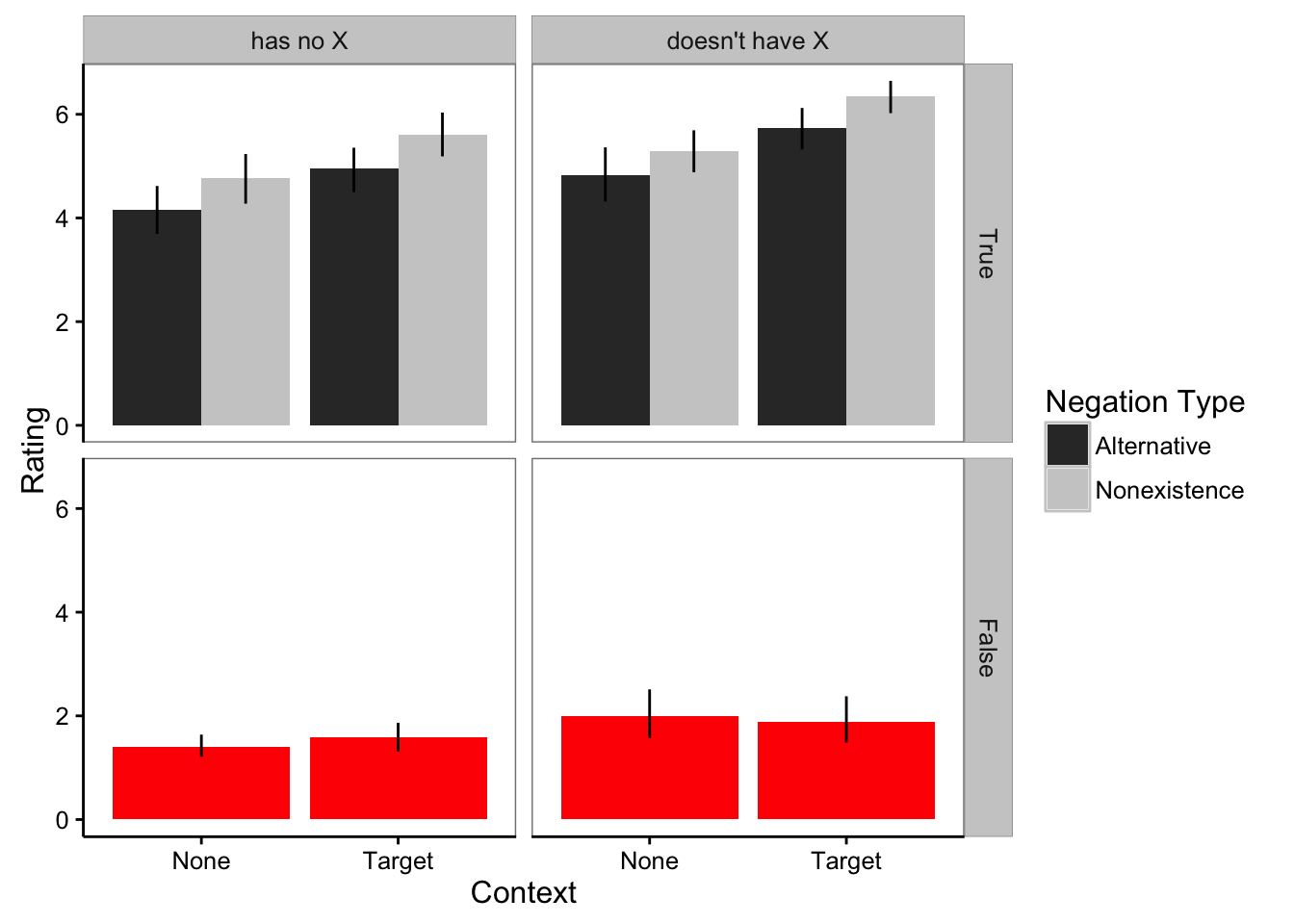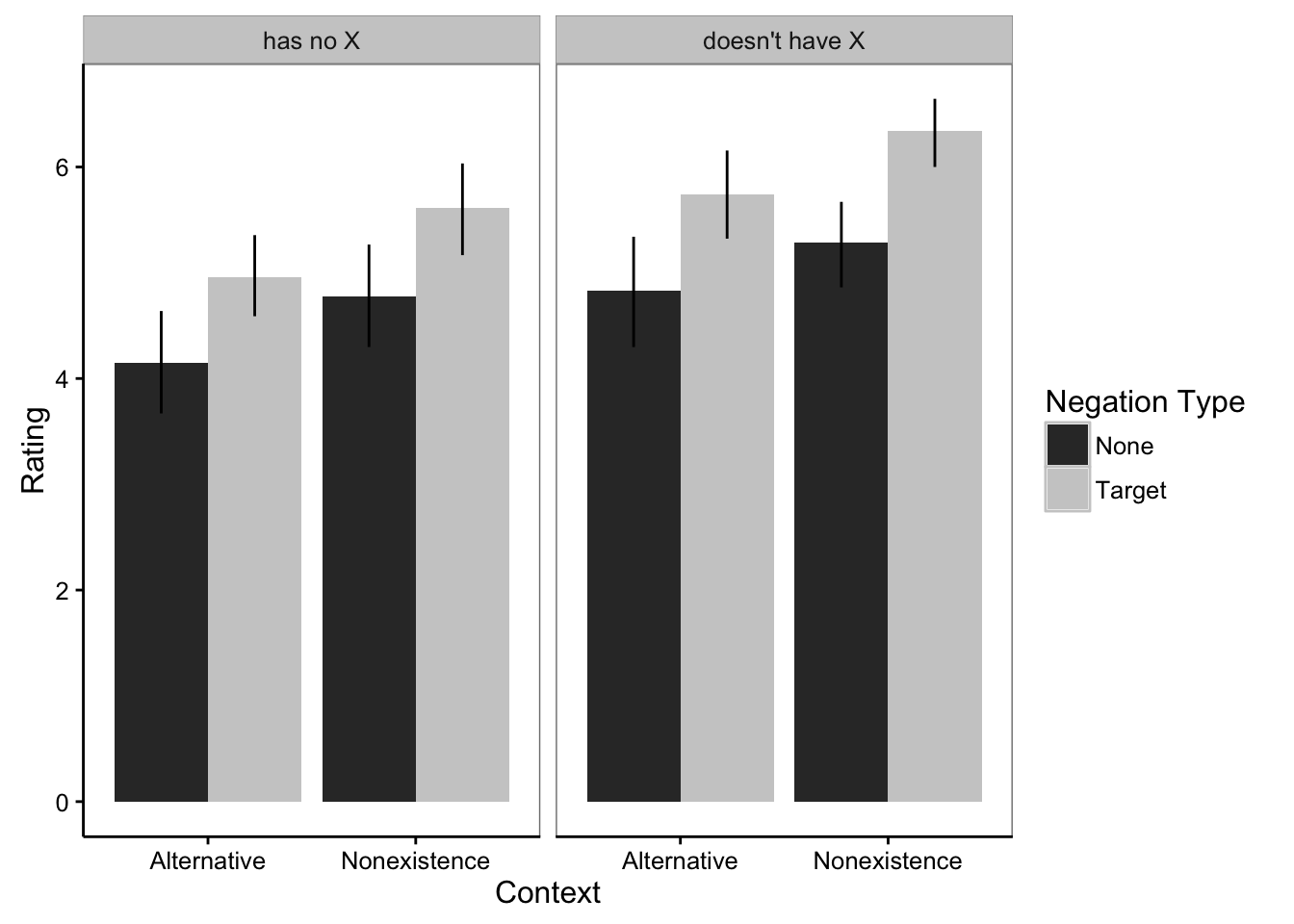Prelims and libraries.

``````rm(list = ls())
library(reshape2)
library(dplyr)``````
``````##
## Attaching package: 'dplyr'
##
## The following objects are masked from 'package:stats':
##
##     filter, lag
##
## The following objects are masked from 'package:base':
##
##     intersect, setdiff, setequal, union``````
``library(ggplot2)``
``## Warning: package 'ggplot2' was built under R version 3.2.3``
``````library(bootstrap)
library(lme4)``````
``## Loading required package: Matrix``
``library(knitr)``

Functions and add some style elements for ggplot2

``````## number of unique subs
n.unique <- function (x) {
length(unique(x))
}

## for bootstrapping 95% confidence intervals
theta <- function(x,xdata) {mean(xdata[x])}
ci.low <- function(x) {
quantile(bootstrap(1:length(x), 1000, theta, x)\$thetastar, .025)}
ci.high <- function(x) {
quantile(bootstrap(1:length(x), 1000, theta, x)\$thetastar, .975)}

plot.style <- theme_bw() +
theme(panel.grid.minor = element_blank(),
panel.grid.major = element_blank(),
legend.position = "right",
axis.line = element_line(colour = "black",size = .5),
axis.ticks = element_line(size = .5),
axis.title.x = element_text(vjust = -.5),
axis.title.y = element_text(angle = 90,vjust = 0.25))``````

## Experiment 1: Adults, between-subjects, referent type & syntactic frame

``````d.exp1 <- read.csv("data/exp1.csv")
d.exp1\$condition <- factor(d.exp1\$condition,
levels = c("noContext", "context"),
labels = c("None", "Target"))
d.exp1\$sentence.type <- factor(d.exp1\$sentence.type,
levels = c("positive", "negative"),
labels = c("Positive" , "Negative"))

d.exp1\$neg.concept <- factor(d.exp1\$neg.concept,
levels = c("something", "nothing"),
labels = c("Alternative",  "Nonexistence"))

d.exp1\$neg.syntax <- factor(d.exp1\$neg.syntax,
levels = c("", "has no", "doesn't have"),
labels = c("", "has no X", "doesn't have X"))``````

### Plot data

Histogram of rating scale:

``qplot(d.exp1\$rating, geom = "histogram") + plot.style``
``## `stat_bin()` using `bins = 30`. Pick better value with `binwidth`.``Plot mean ratings for every trial type (Table 4 in paper):

``````ms <- d.exp1 %>%
group_by(subid, condition, sentence.type, truth.value, neg.concept, neg.syntax) %>%
summarise(subm = mean(rating)) %>%
group_by(condition, sentence.type, truth.value, neg.concept, neg.syntax) %>%
summarise(rating = mean(subm),
high = ci.high(subm),
low = ci.low(subm))
ms\$truth.value <- factor(ms\$truth.value, levels=c(TRUE, FALSE), labels=c("True","False"))

kable(ms, digits = 2)``````
condition sentence.type truth.value neg.concept neg.syntax rating high low
None Positive False Alternative 2.04 2.60 1.57
None Positive False Nonexistence 2.51 3.06 1.96
None Positive True NA 6.51 6.73 6.22
None Negative False NA has no X 1.40 1.66 1.19
None Negative False NA doesn’t have X 2.00 2.51 1.57
None Negative True Alternative has no X 4.15 4.68 3.65
None Negative True Alternative doesn’t have X 4.83 5.33 4.29
None Negative True Nonexistence has no X 4.78 5.20 4.32
None Negative True Nonexistence doesn’t have X 5.29 5.69 4.85
Target Positive False Alternative 1.83 2.38 1.36
Target Positive False Nonexistence 1.88 2.31 1.49
Target Positive True NA 6.42 6.63 6.20
Target Negative False NA has no X 1.58 1.87 1.31
Target Negative False NA doesn’t have X 1.89 2.38 1.44
Target Negative True Alternative has no X 4.96 5.34 4.54
Target Negative True Alternative doesn’t have X 5.74 6.10 5.32
Target Negative True Nonexistence has no X 5.61 6.02 5.19
Target Negative True Nonexistence doesn’t have X 6.34 6.63 5.99
``````ggplot(data = ms, aes(x = sentence.type, y = rating,
color = neg.concept, shape = neg.syntax)) +
geom_point(stat = "identity", position = position_dodge(width = .5)) +
facet_grid(truth.value ~ condition) +
geom_errorbar(aes(ymin = low, ymax = high), width = 0,
position = position_dodge(width = .5)) +
plot.style``````Just look at negative sentences:

``````d.exp1.neg <- filter(d.exp1, sentence.type == "Negative")

ms <- d.exp1.neg %>%
group_by(subid, condition, truth.value, neg.concept, neg.syntax) %>%
summarise(subm = mean(rating)) %>%
group_by(condition, truth.value, neg.concept, neg.syntax) %>%
summarise(rating = mean(subm),
high = ci.high(subm),
low = ci.low(subm))
ms\$truth.value <- factor(ms\$truth.value, levels=c(TRUE, FALSE), labels=c("True","False"))

ggplot(data = ms, aes(x = condition, y = rating, fill = neg.concept)) +
geom_bar(stat = "identity", position = "dodge") +
facet_grid(truth.value ~ neg.syntax) +
geom_errorbar(aes(ymin = low, ymax = high), width = 0,
position = position_dodge(width = .9)) +
scale_fill_grey("Negation Type") +
xlab("Context") + ylab("Rating") +
plot.style``````Just look at true negatives (Figure 2 in paper)

``````d.exp1.trueneg <- filter(d.exp1, sentence.type ==  "Negative" & truth.value == TRUE)

ms <- d.exp1.trueneg %>%
group_by(subid, condition, neg.concept, neg.syntax) %>%
summarise(subm = mean(rating)) %>%
group_by(condition, neg.concept, neg.syntax) %>%
summarise(rating = mean(subm),
high = ci.high(subm),
low = ci.low(subm))

ggplot(data = ms, aes(x = neg.concept, y = rating, fill = condition)) +
geom_bar(stat = "identity", position = "dodge") +
facet_wrap(~ neg.syntax) +
geom_errorbar(aes(ymin = low, ymax = high), width = 0,
position = position_dodge(width = .9)) +
scale_fill_grey("Negation Type") +
xlab("Context") + ylab("Rating") +
plot.style``````### Statistical models:

All data:

``````#deviation coding
contrasts(d.exp1\$sentence.type) <- rbind(.5, -.5)
contrasts(d.exp1\$sentence.type)``````
``````##          [,1]
## Positive  0.5
## Negative -0.5``````
``````contrasts(d.exp1\$condition) <- rbind(.5, -.5)
contrasts(d.exp1\$condition)``````
``````##        [,1]
## None    0.5
## Target -0.5``````
``````contrasts(d.exp1\$truth.value) <- rbind(.5, -.5)
contrasts(d.exp1\$truth.value)``````
``````##       [,1]
## FALSE  0.5
## TRUE  -0.5``````
``````model.exp1.all <- lmer(rating ~ sentence.type * condition * truth.value +
(sentence.type * truth.value | subid) +
(sentence.type * truth.value | item),
data = d.exp1)

kable(summary(model.exp1.all)\$coefficients, digits = 2)``````
Estimate Std. Error t value
(Intercept) 3.87 0.07 51.78
sentence.type1 0.80 0.08 9.60
condition1 -0.11 0.14 -0.79
truth.value1 -3.94 0.18 -21.96
sentence.type1:condition1 0.71 0.17 4.23
sentence.type1:truth.value1 -0.89 0.21 -4.24
condition1:truth.value1 0.59 0.35 1.68
sentence.type1:condition1:truth.value1 -0.57 0.40 -1.43

Just negative sentences:

``````contrasts(d.exp1.neg\$condition) <- rbind(.5, -.5)
contrasts(d.exp1.neg\$condition)``````
``````##        [,1]
## None    0.5
## Target -0.5``````
``````contrasts(d.exp1.neg\$truth.value) <- rbind(.5, -.5)
contrasts(d.exp1.neg\$truth.value)``````
``````##       [,1]
## FALSE  0.5
## TRUE  -0.5``````
``````model.exp1.neg <- lmer(rating ~ condition * truth.value +
(truth.value | subid) +
(condition * truth.value | item),
data = d.exp1.neg)

kable(summary(model.exp1.neg)\$coefficients, digits = 2)``````
Estimate Std. Error t value
(Intercept) 3.46 0.08 44.45
condition1 -0.46 0.16 -2.89
truth.value1 -3.49 0.21 -16.70
condition1:truth.value1 0.86 0.41 2.09

Just true negative sentences (Table 1 in paper):

``````contrasts(d.exp1.trueneg\$condition) <- rbind(.5, -.5)
contrasts(d.exp1.trueneg\$condition)``````
``````##        [,1]
## None    0.5
## Target -0.5``````
``````contrasts(d.exp1.trueneg\$neg.concept) <- rbind(.5, -.5)
contrasts(d.exp1.trueneg\$neg.concept)``````
``````##              [,1]
## Alternative   0.5
## Nonexistence -0.5``````
``````d.exp1.trueneg\$neg.syntax <- factor(d.exp1.trueneg\$neg.syntax)
contrasts(d.exp1.trueneg\$neg.syntax) <- rbind(.5, -.5)
contrasts(d.exp1.trueneg\$neg.syntax)``````
``````##                [,1]
## has no X        0.5
## doesn't have X -0.5``````
``````model.exp1.trueneg <- lmer(rating ~ condition * neg.concept * neg.syntax +
(neg.concept * neg.syntax | subid) +
(neg.concept * neg.syntax | item),
data = d.exp1.trueneg)

kable(summary(model.exp1.trueneg)\$coefficients, digits = 2)``````
Estimate Std. Error t value
(Intercept) 5.21 0.13 38.78
condition1 -0.90 0.26 -3.45
neg.concept1 -0.60 0.12 -5.05
neg.syntax1 -0.68 0.17 -3.99
condition1:neg.concept1 0.11 0.19 0.55
condition1:neg.syntax1 0.15 0.29 0.52
neg.concept1:neg.syntax1 -0.11 0.18 -0.64
condition1:neg.concept1:neg.syntax1 -0.22 0.32 -0.67

## Experiment 2: Children, between subjects, none vs. target context

``````d.exp2 <- read.csv("data/exp2.csv")

d.exp2\$agegroup <- factor(d.exp2\$agegroup)

d.exp2\$sent.type <- factor(d.exp2\$sent.type,
levels = c("positive", "negative"),
labels = c("Positive", "Negative"))

d.exp2\$condition <- factor(d.exp2\$condition,
labels = c("None", "Target"))``````

Condense scale from 5-point to 3-point

``````d.exp2\$resp2 <- 2
d.exp2[d.exp2\$resp > 3,]\$resp2 <- 3
d.exp2[d.exp2\$resp < 3,]\$resp2 <- 1
d.exp2\$resp2 <- factor(d.exp2\$resp2)``````

### Participant exclusions

Exclude subjects who didn’t complete at least half of the trials

``````#Reject children who didn't complete at least 8 trials
ntrials <- d.exp2 %>%
group_by(subid) %>%
summarize(ntrials = n()) %>%
filter(ntrials < 8)

for (i in ntrials\$subid) {
d.exp2 <- filter(d.exp2, subid != i)
}``````

Reject subjects who don’t understand scale (based on positive sentences):

``````reject <- d.exp2 %>%
filter(sent.type == "Positive") %>% #Only look at positive sentences
group_by(subid) %>%
mutate(total = n()) %>% #get total # of positive sentences child saw
group_by(subid, condition, truth, total, resp2) %>%
filter((truth == "True" & resp2 == 3) | (truth == "False" & resp2 == 1)) %>% #Get # "good" for true pos and "bad" for false pos
summarize(counts = n()) %>%
group_by(subid, condition, total) %>%
summarize(counts = sum(counts)) %>% #total # "correct" responses
mutate(prop = counts/total) %>% #proportion correct
filter(prop < .6) #reject kids who got < .6 "correct" (this allows for 2/6 "mistakes")

for (i in reject\$subid) {
d.exp2 <- filter(d.exp2, subid != i)
}``````

Make sure there aren’t any kids who just used one side of scale. Reject kids who only chose a single data point

``````scaleUse <- aggregate(resp2 ~ subid, d.exp2, n.unique)
table(scaleUse\$resp2) #Are any resp2 = 1``````
``````##
##  2  3
## 48 21``````

### Plot data, all ages

Categorize kids based on response type

``````tn_responses <- d.exp2 %>%
filter(sent.type == "Negative" & truth == "True") %>%
group_by(subid) %>%
mutate(total = n()) %>%
group_by(subid, condition, total, resp2) %>%
summarize(counts = n()) %>%
mutate(prop = counts/total)

category <- dcast(tn_responses, subid + condition ~ resp2)``````
``## Using prop as value column: use value.var to override.``
``````names(category) <- c("subid","condition","bad","neutral","good")
category[is.na(category)] <- 0

category\$type <- "other"
#category[category\$neutral > .6,]\$type <- "tn_neutral"